# Lesson 6: Properties of electrons and the quantum mechanical measurement process

## Properties of electrons and the quantum mechanical measurement process

6.1 The property “position” in the double-slit experiment – 6.2 Measurement process and complementarity – 6.3 Measurement and properties 6.4 State reduction
6.5 Schrödinger’s cat, measurement process and decoherence – 6.6 Progress check – 6.7 Summary

This chapter puts the focus on further “strange features” of quantum mechanics. Using the example of the double-slit experiment, we show that an electron generally cannot be assigned the property “position”.

Furthermore, we discuss the fact that the measurement process plays a very unique and important role in quantum mechanics. The paradox of Schrödinger’s cat represents a further example for the strangeness of quantum mechanics.

### 6.1 The property “position” in the double-slit experiment

If we consider the behavior of electrons at the double slit, we come to the following conclusion: According to the classical idea, around half the electrons pass through slit 1 and the other half through slit 2. According to this view, each particle passes through precisely one specific slit (we just do not know through which one). But with the corresponding experimental effort it would be possible to accurately determine exactly which slit (= position) the particle passes through. By detecting its point of impact on a screen behind the slit, the complete path of the particle can be reconstructed, even predicted, when the initial conditions (e. g. the momentum) are known.

If we were to now close one slit, this would not have a negative impact on the behavior of the particles which would pass through the other slit anyway (Fig. 6.1 a). This would be exactly the same the other way round (Fig. 6.1. b). The overall image on the screen, i. e. the impact probability, would remain unaffected by this: The distribution would result simply from the sum of the single slit distributions: P(x) = P1(x) + P2(x), (Fig. 6.1 c). How would the particles which passed through one slit “know” whether the other slit was closed or open? We already know the result from Chapter 5.4. This is the concept of classical mechanics.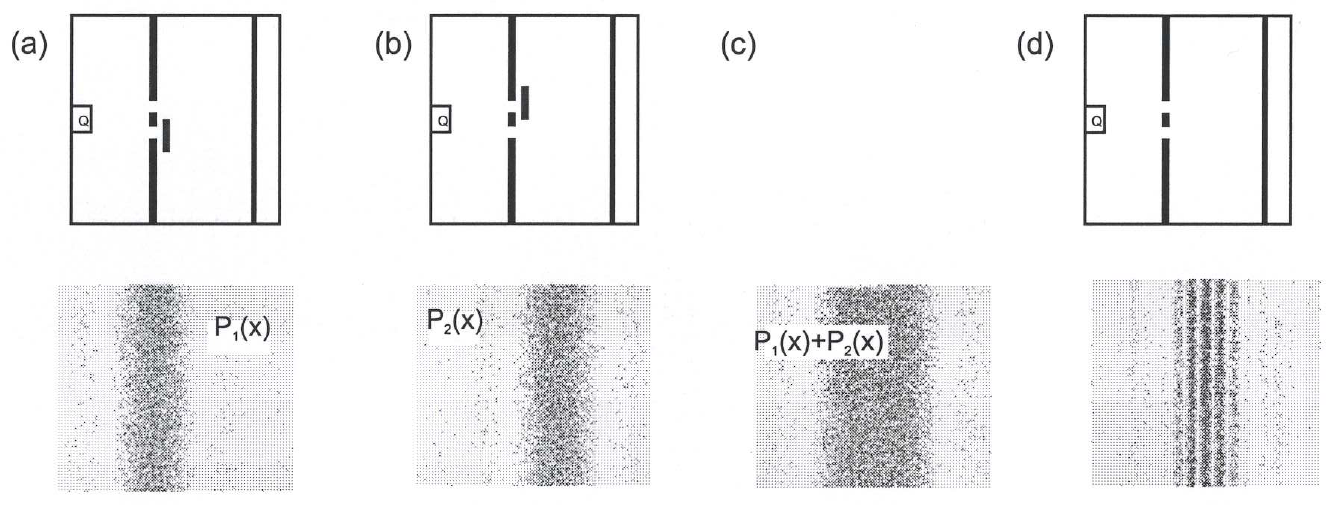Fig. 6.1: Property “position” in the double-slit experiment

According to quantum mechanics, this concept is wrong, however. The double-slit distribution with electrons (in general with quantum objects) does indeed exhibit an interference pattern (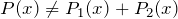, Fig. 6.1 d), which cannot be explained by the fact that every electron passes through a specific slit, i. e. it can always be localized. On the contrary, we are forced to abandon the concept of electrons as localized entities completely. It is not only that we do not know the slit through which the electron could have gone – that would imply that a slit, i.e. a path (position), could in principle be assigned to electrons, but we do not know which one it is (see above). No – the property “position” cannot be assigned to an electron. Therefore, a quantum object does not possess the property “position” in the slit plane. This is a radical departure from the classical concepts.

In summary, this means:

In quantum mechanics, it is possible that classically well-defined properties cannot be assigned to a quantum object. For example, an electron in the double-slit experiment is at no time at a specific position as it propagates “unobserved” through space, i. e. it does not possess the property “position”.

We already found something similar in Chapter 3.4: It was shown that the property “path” cannot be assigned to a photon in the Mach-Zehnder interferometer without creating contradictions.

### 6.2 Measurement process and complementarity

We explained in the last section that the property “position” (for example with the double slit) cannot be assigned to a quantum object.

In the classical sense, it appears to be paradoxical that we can definitely carry out a position measurement on an electron and obtain a result as well. As a reminder, it should be said that a position measurement describes a typical particle property in classical physics. In contrast, the interference pattern on a screen after passing through a double slit is – for electrons as well – a typical wave phenomenon.

A simulation experiment (Experiment 6.1 in the teaching materials) in the double-slit program shows how a position measurement could be realized:
A lamp, whose light scatters off electrons and thus makes them detectable (light flash), is switched on between screen and double slit. Cf Fig. 6.2.1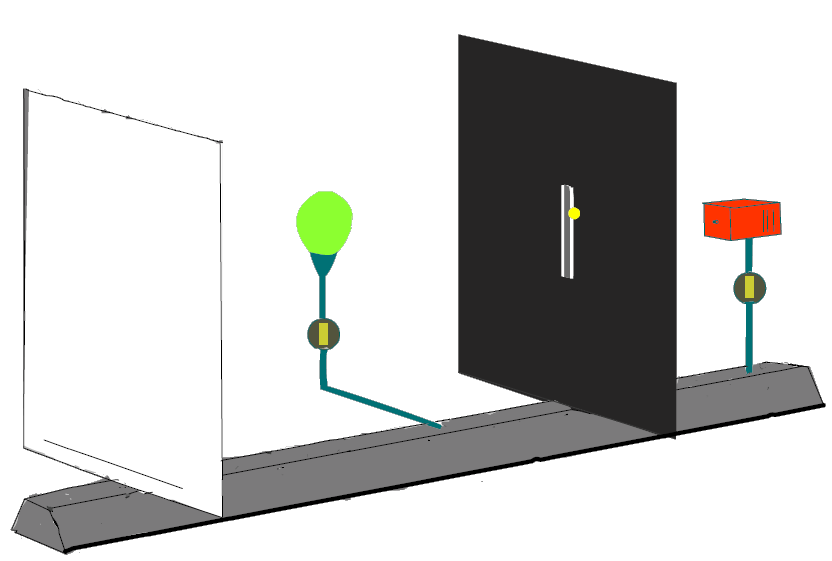Fig. 6.2.1

Each electron is found at a well-defined position in such a position measurement, and there is a high probability that it is behind one of the two slits, although behind which one exactly cannot be stated in advance. So far, so good, but with this experimental arrangement we will find out that it is no longer possible to recognize an interference pattern – instead the sum of the two single slit distributions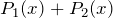turns up again.

We see from the result of Experiment 6.1 that we cannot outwit quantum mechanics. Although we find an electron behind precisely one of the two slits in a position measurement, it is now a different experiment according to Bohr. This is expressed by the fact that no double-slit interference pattern appears, for example. Experimental arrangements where wave properties (interference) occur are complementary to those where the “particle-like” behavior (position determination) of the quantum objects is recorded. Complementary arrangements cannot be realized by a single experimental arrangement.

The property position and the interference cannot be realized simultaneously, but are mutually exclusive. This is a special case of a general principle which is called complementarity as suggested by Nils Bohr.

The complementarity between interference pattern and path information can be investigated in more detail with the aid of Experiment 6.2. The intensity of the lamp is varied here so that it is no longer possible to detect all the electrons at low intensity. Those not detected contribute for their part to the emergence of the interference pattern again, however. The other, “localized” electrons produce a distribution without any structure. The transition is fluent. We have already come across similar behavior by photons in the Mach-Zehnder interferometer with polarization filters, and the quantum eraser experiment (Chap. 3.4).

We see from the experiments that changing even a small part of the experimental set-up is sometimes sufficient to qualitatively change the experimental result. Bohr calls this the completeness of quantum phenomena.

In quantum physics, the result of experiments has a very sensitive dependence on the experimental set-up.

Bohr’s interpretation of quantum mechanics (the so-called Copenhagen interpretation) is very complex. Here you can download an article which explains the Bohr philosophy in more detail (published in “Physik in der Schule” 34 (1996), pp.165 – 170).

### 6.3 Measurements and properties

Measurement in classical physics:

In classical physics, the measurement of a quantity simply means noting its value, which is already well defined and fixed. By positioning a yardstick, for example, we can easily determine the impact point of a ball thrown from a specific launch point. The position of the ball here is obviously independent of whether a measurement is made at all or not, and for a repeat measurement, the ball would naturally be found at the same position (albeit under the constraint of statistical measurement errors (spread)).

Measurement in quantum mechanics:

As we have seen (Section 6.1), quantum objects can sometimes not be assigned the property position at all (e. g. in the slit plane (Chapter 5.4) or with the Mach-Zehnder interferometer (Chapter 3.4)) (this also applies to other properties such as the momentum or the energy of a quantum object) – it therefore does not possess this property at all. What is the result of a position measurement when the quantum object does not possess the property position? What does a measuring instrument measure in these cases?

In fact, all measurements in real experiments show an unequivocal value of the measured quantity! Even in the simulation experiment in Section 6.2, a flash of light lights up at only one single position behind the double slit during the position measurement. In the measurement, each electron is therefore found behind one of the slits, although it would be wrong to claim that it had already possessed the property “is behind one of the slits” before the measurement. This means that, of the possible measurement values (slit 1 or slit 2), precisely one has been selected in the measurement. If the same experiment is carried out several times in succession, different results will be obtained in general. In the example considered, the electron will be found as often behind slit 1 as behind slit 2 on average, if both slits are irradiated uniformly. This yields the:

Measurement postulate of quantum mechanics:

In each measurement on a quantum object, one single measurement value from the range of possible values (here slit 1 or slit 2) is realized. The probability with which a measured value is found can be determined from the wave function by means of the Born probabilistic equation.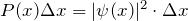Measurement postulate of quantum mechanics

Heisenberg on the measurement process

The discussion regarding the measurement postulate shows:
From the fact that a certain value was obtained for the measurement of a position, we may not conclude that the quantum object possessed this property beforehand. There is therefore no “path” either (i. e. no sequence of positions) on which the electron moves from the source to the screen, but only a quantum mechanical wave function which propagates according to the laws of wave mechanics. One of the two possibilities is realized only in the measurement process.

This clearly illustrates yet again that a quantum object does not have to possess a specific property (such as position) at all. If a measurement is carried out, however, a measured value is always found, although the quantum object did not exhibit this property beforehand.

In quantum mechanics, there is a difference between “possessing a property” and “measuring a property”.

The two statements below are not equivalent, for example:

a) In a measurement, the electron is found to be at position x.

b) An electron possesses the property “position x”.

Here the ensemble definition introduced in Chapter 4 turns out to be useful. Measurements on the individual quantum objects of an ensemble provide an unequivocal answer as to whether a specific property can be assigned to quantum objects or not:

1. The measured values have a spread, i. e. in some measurements, the electron is found behind “slit 1”, in others behind “slit 2”. In this case, the ensemble behind the slits does not have the property “position”.

2. All measurements provide the same position (within a certain interval), e. g. “slit 1”. The ensemble then also has the property “position”. In this case (and only in this case) we can also say that each individual member of the ensemble possesses the property concerned.

Dynamic properties of quantum objects therefore always relate to a whole ensemble (as does the concept of preparation).

6.4 State reduction

Every physical measurement is a process whereby physical measuring instruments interact with the object to be observed (otherwise there would be no information on the object). The measurement itself affects the quantity to be measured as well (like a thermometer changes the temperature of a liquid in which it is immersed). In classical physics, these measurement effects are either negligible or can be compensated with the aid of theory.

Measurements in quantum physics represent a non-negligible intervention in the undisturbed course of events; they change the state of the object measured! An example here is the double-slit experiment with measurement light source familiar from Section 6.2. When the position measurement is carried out – i. e. when the measurement lamp is switched on – an interference pattern no longer emerges on the screen. After the measurement, the electrons are obviously in a different state which is not able to interfere. The measurement has changed the state of the electrons.  This is a general characteristic of measurements in quantum mechanics:

In contrast to classical physics, a quantum mechanical measurement changes the state of the system on which the measurement is made.

The phenomenon can be discussed quantitatively with the aid of the wave function:

The wave function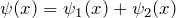describes the state of the system with the light source switched off. It is a superposition state of the two wave functions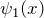and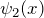(Fig. 6.3.1). The probability distribution of the electrons on the screen is given by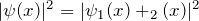(cf. Chapters 5.3 and 5.4). The interference pattern is obtained.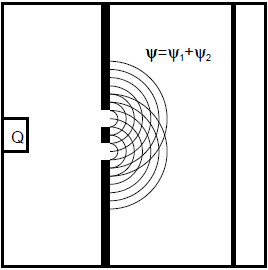Fig. 6.3.1

The measurement – a position measurement takes place when the measurement lamp is switched on – selects one of the two possibilities; the electrons are in theorstate after the measurement, depending on the result of the position measurement (cf. Fig. 6.3.2: Here the electrons found behind slit 1 (light flash = position measurement) are described by the single-slit wave function. Their contribution to the electron distribution on the screen corresponds to the single-slit distribution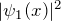. The same applies to slit 2.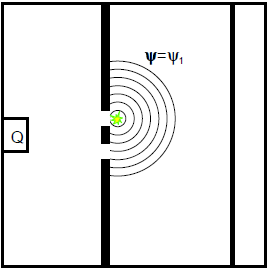Fig. 6.3.2

The pattern which ultimately forms on the screen is the sum of the two single-slit distributions; it is characterized by the lack of an interference structure: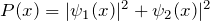. The fact that no interference pattern is obtained in this experiment is therefore explained by the fact that the electrons are described by a different wave function after the measurement.

The transition from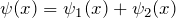to one of the two possibilitiesoris called state reduction or “collapse” of the wave function. The state reduction represents a sudden change in the wave function which is characteristic for a measurement.

The discussion on the interpretation of the state reduction stretches from the very first days of quantum mechanics through to the present. It is one of the most hotly disputed topics in quantum mechanics.

The corresponding wave functions or probability distributions before and after the measurement are summarized again in the table below:

 Before the measurement After the measurement Wave function: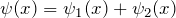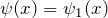oder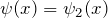Probability: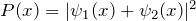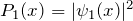oder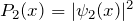### 6.5 Schrödinger’s cat, measurement process and decoherence

We do not notice any strange quantum behavior with the phenomena we experience in daily life. The question is whether quantum mechanics can explain how the world assumes its “classical” manifestation when we go from microscopic to macroscopic systems.

“Schrödinger’s cat” is a famous paradox of quantum mechanics, the trigger for the question as to whether quantum mechanics has a classical limit case in which it reproduces the statements of classical physics.
Superposition states like those we met with the double-slit experiment do not occur in macroscopic physics. To illustrate this, Schrödinger designed his cat paradox.

Schrödinger writes:

“One can even set out quite ridiculous cases. A cat is penned up in a steel chamber, along with the following diabolical device (which must be secured against direct interference by the cat): in a Geiger counter, there is a tiny bit of radioactive substance, so small, that in the course of an hour perhaps one of the atoms decays, but also, with equal probability, perhaps none; if it happens, the counter tube discharges and through a relay releases a hammer that shatters a small flask of hydrocyanic acid. If one has left this entire system to itself for an hour, one would say that the cat still lives if meanwhile no atom has decayed. The first atomic decay would have poisoned it. The Ψ function of the entire system would express this by having in it the living and dead cat mixed or smeared out in equal parts.

It is typical of these cases that an indeterminacy originally restricted to the atomic domain becomes transformed into macroscopic indeterminacy, which can then be resolved by direct observation. That prevents us from so naively accepting as valid a “blurred model” for representing reality.”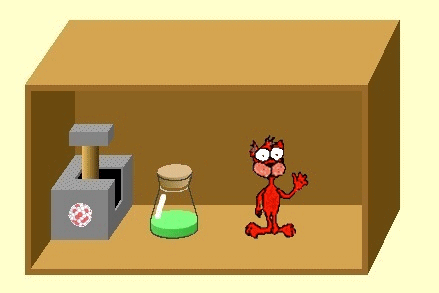According to Schrödinger, the wave function of the whole system (chamber + cat) has the form of a superposition state of two macroscopically different states after one hour has passed:,

wheredescribes the state “atom decayed and cat dead” andthe state “atom not decayed and cat alive”. As was the case with the double-slit experiment, the wave function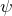has the form of a superposition state. In analogy with the considerations of the property position, this means the cat does not possess the property “dead” or “alive”. Schrödinger thus succeeded with this example in illustrating that the transition from quantum mechanics to classical physics cannot be managed without difficulties.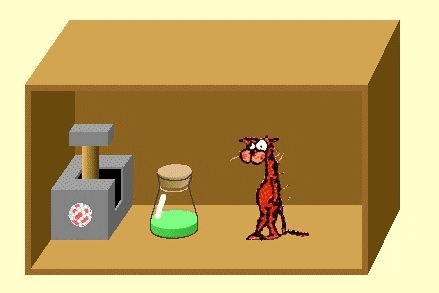The quantum mechanical measurement problem

Instead of the cat, we can consider a measuring instrument that is to indicate the decay of an atom in the radioactive substance by a lamp lighting up. Here as well, quantum mechanics predicts that in a measurement, the measuring instrument does not indicate an unequivocal value, because the system is in a superposition state (lamp is lit up and is not lit up simultaneously). This also contradicts all our experiences with measuring instruments.

To obtain agreement with the observed behavior of measuring instruments, the abrupt process of state reduction (Section 6.4) was introduced “manually”: The act of taking a measurement “reduces” the wave function from the superposition state to one of the possibilities (“lamp is lit” / ”lamp is not lit”) according to the laws of probability (cf. also collapse of the wave function; Section 6.4).

Decoherence

In recent years, progress has been made in arriving at a solution for the cat paradox. The theory of decoherence explains why no superposition states are registered in the macroscopic world. The key idea here is that macroscopic bodies (such as the cat) cannot be considered in isolation. They must be considered as open systems interacting with the outside world. They always have a natural environment with which they interact in various ways. The cat scatters light, emits thermal radiation, and has an impact on the air molecules in its vicinity, for example. By interacting with its natural environment, the cat becomes “effectively classical”. It is dead or alive; superpositions or interference phenomena cannot be detected. The interaction with the environment destroys the ability to interfere.

There are also special cases where isolation from the environment is possible, however. No decoherence then takes place and macroscopic quantum phenomena such as superconductivity, superfluidity and Bose-Einstein condensation occur.

### 6.6 Progress check

The following points were important in this chapter:

• There are cases where classically well-defined properties (e. g. position) cannot be assigned to a quantum object.
• The concept of complementarity and the principle of the completeness of quantum phenomena.
• Measurement process and measurement postulate.
• State reduction.
• The Schrödinger cat paradox and its meaning.

Before you move on to the next chapter, make sure you know the fundamental ideas behind these points. You can then check this with the aid of the Summary.

### 6.7 Summary

A key characteristic of quantum mechanics is that classically well-defined properties (e. g. position) possibly cannot be assigned to a quantum object.

The property position and the interference pattern cannot be realized simultaneously, but are mutually exclusive. This is a special case of a more general principle which is called complementarity as suggested by Nils Bohr.

A certain value is found in each measurement of a physical observable (measurement postulate). There is therefore a difference between “possessing a property” and “measuring a property”.

The transition from the wave function Ψ(x) to one of the two wave functionsor(corresponding to the measured value) is called state reduction. State reduction is a sudden change of the wave function.

The Schrödinger’s cat paradox concerns the transition from quantum mechanics to macroscopic physics. The problem is the existence of superposition states, which are foreign to classical mechanics. The theory of decoherence explains why no superpositions of dead and living cats are observed in the macroscopic world.